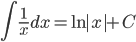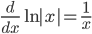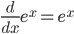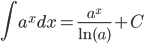### Day 71 - Differentiation of Logarithms - 04.29.15

Update
• Unit 5 Test, this Friday!

Questions

Bell Ringer
• Posted on the board!

Review
• Prerequisites
• Derivatives
• Derivative Rules
• Power Rule
• Constant Rule
• Trig Derivatives
• Logarithm Properties
• Differentiation using the Natural Logarithmic Functions (video)
• Integration using the Natural  Logarithmic Function (video)
• Exponential Functions (e^x): Differentiation and Integration (video)
• Exponential Functions (a^x): Differentiation and Integration (video)

Lesson
• Exponential Functions (a^x): Differentiation and Integration (video)
•Exit Ticket
• Posted on the board at the end of the block!

Lesson Objective(s)
• How can the derivative of logarithmic functions be calculated?
Skills
1. Calculate the derivative of the logarithmic function.

#### In-Class Help Requests

Standard(s)
• APC.9
• Apply formulas to find derivatives.
• Includes:
• derivatives of algebraic, trigonometric, exponential, logarithmic, and inverse trigonometric functions
• derivations of sums, products, quotients, inverses, and composites (chain rule) of elementary functions
• derivatives of implicitly defined functions
• higher order derivatives of algebraic, trigonometric, exponential, and logarithmic, functions